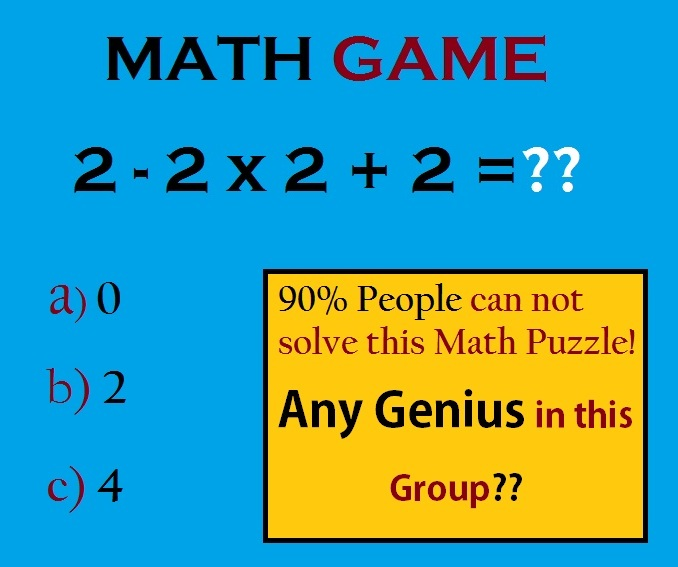This post may contain affiliate links. This means if you click on the link and purchase the item, We will receive an affiliate commission at no extra cost to you. See Our Affiliate Policy for more info.

# 2-2×2+2 =?? Basic but Tricky Math Puzzles with Answers

8
13499

Hello Peoples, Here’s an interesting math puzzle you to solve. Let see how’s your basic math is?

90% People can not solve this Basic Math Puzzle Problem. Any Genius are there to solve this?

## MATH GAME :   2 – 2 x 2 + 2 = ??

Three options are given, Choose the Correct one.

And of course if you can’t, the answer & solution given below.

# Can You Solve this Math Puzzle?Solve it?? Let’s see you are correct or not? See you in comments! 😀

Solution :-

2 – 2 x 2 + 2 = ??

According to “PMDAS” rule…

First Multiplication then, Division, Addition, Subtraction.

2 – 2 x 2 + 2 = ?

2 – 4 + 2 = ?

2 – 2 = 0

Search Items:-

Math Puzzles, Math Game, Math Riddles, Math Puzzles with Answers, Only For Genius Math Puzzles, 2-2×2+2 =? Math Puzzles with Answer, Math Brainteaser, Brain Games, Tricky Math Puzzles, Puzzles and Riddles, Puzzles For Whats App, Puzzles For Facebook, Tricky Riddles, Hard Math Puzzles, Tough Math Puzzles, Pics Story

1.Edward Tazelaar

I see some people don’t remember basic math order. Multiplication and division is done before addition and subtraction if there are no parenthesis. The answer is 0. 2 – (2×2) + 2, 2-4+2=0

2.Anwar

So it’s 4

3.Neha

Ans is 4

4.Dheeru

Ans is 0.

5.Vaishu

0 is answer apdina first 2* 2=4then 2-4+2=-2+2=0its simple

6.Hussain Shaikh

7.Dharatri behera
8.Pop-Corn